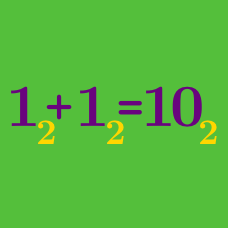Number Theory

Number Bases: Level 1 Challenges

A $3$-digit number has digit sum $15$ and is divisible by $35$. Find the $3$-digit number.

Convert the following number from base 3 to base 9: $\huge{121212121212_3}$

$1111111111111111_{(2)} + 1010101010101010_{(2)} = \ ?$

Clarification: The two numbers given are written in base 2, while the numbers in the answer choices are written in base 16.

True or false:

$\quad$ $\large 0.{111111\ldots}_2 = 1$.

Evaluate the following in base 10 :

$100_2+ 100_{8}+ 100_{10} + 100_{16}$

×